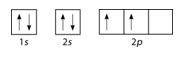Electron Configurations

Learning Objectives

• Use electron configuration notation to indicate the electron configuration of an atom.

How big is a file?

If you keep your papers in manila folders, you can pick up a folder and see how much it weighs. If you want to know how many different papers (articles, bank records, or whatever else you keep in a folder), you have to take everything out and count. A computer directory, on the other hand, tells you exactly how much you have in each file. We can get the same information on atoms. If we use an orbital filling diagram, we have to count arrows. When we look at electron configuration data, we simply add up the numbers.Electron configuration notation eliminates the boxes and arrows of orbital filling diagrams. Each occupied sublevel designation is written followed by a superscript that is the number of electrons in that sublevel. For example, the hydrogen configuration is 1s1, while the helium configuration is 1s2. Multiple occupied sublevels are written one after another. The electron configuration of lithium is 1s22s1. The sum of the superscripts in an electron configuration is equal to the number of electrons in that atom, which is in turn equal to its atomic number.

Sample Problem: Orbital Filling Diagrams and Electron Configurations

Draw the orbital filling diagram for carbon and write its electron configuration.

Step 1: List the known quantities and plan the problem.

Known

• atomic number of carbon, Z = 6

Use the order of fill diagram to draw an orbital filling diagram with a total of six electrons. Follow Hund’s rule. Write the electron configuration.

Step 2: Construct DiagramOrbital filling diagram for carbon.

Electron configuration 1s22s22p2

Following the 2 s sublevel is the 2p, and p sublevels always consist of three orbitals. All three orbitals need to be drawn even if one or more is unoccupied. According to Hund’s rule, the sixth electron enters the second of those p orbitals and with the same spin as the fifth electron.

Second Period Elements

Periods refer to the horizontal rows of the periodic table. Looking at a periodic table you will see that the first period contains only the elements hydrogen and helium. This is because the first principal energy level consists of only the s sublevel and so only two electrons are required in order to fill the entire principal energy level. Each time a new principal energy level begins, as with the third element lithium, a new period is started on the periodic table. As one moves across the second period, electrons are successively added. With beryllium (Z = 4), the 2s sublevel is complete and the 2p sublevel begins with boron (Z = 5). Since there are three 2 p orbitals and each orbital holds two electrons, the 2p sublevel is filled after six elements. The Table below shows the electron configurations of the elements in the second period.

 Element Name Symbol Atomic Number Electron Configuration Lithium Li 3 1s22s1 Beryllium Be 4 1s22s2 Boron B 5 1s22s22p1 Carbon C 6 1s22s22p2 Nitrogen N 7 1s22s22p3 Oxygen O 8 1s22s22p4 Fluorine F 9 1s22s22p5 Neon Ne 10 1s22s22p6

Summary

• Electron configuration notation simplifies the indication of where electrons are located in a specific atom.
• Superscripts are used to indicate the number of electrons in a given sublevel.

Practice

Use the link below to practice solving electron configuration problems.

http://www.sciencegeek.net/Chemistry/taters/Unit2ElectronNotations.htm

Review

1. What does electron configuration notation eliminate?
2. How do we know how many electrons are in each sublevel?
3. An atom has the electron configuration of 1s22s22p5. How many electrons are in that atom?
4. Which element has the electron configuration of 1s22s22p63s2?

Glossary

• electron configuration notation: Each occupied sublevel designation is written followed by a superscript that is the number of electrons in that sublevel.